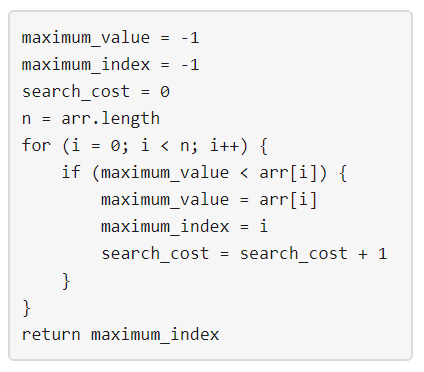##### Welcome to Subscribe On Youtube

Formatted question description: https://leetcode.ca/all/1420.html

# 1420. Build Array Where You Can Find The Maximum Exactly K Comparisons (Hard)

Given three integers n, m and k. Consider the following algorithm to find the maximum element of an array of positive integers:You should build the array arr which has the following properties:

• arr has exactly n integers.
• 1 <= arr[i] <= m where (0 <= i < n).
• After applying the mentioned algorithm to arr, the value search_cost is equal to k.

Return the number of ways to build the array arr under the mentioned conditions. As the answer may grow large, the answer must be computed modulo 10^9 + 7.

Example 1:

Input: n = 2, m = 3, k = 1
Output: 6
Explanation: The possible arrays are [1, 1], [2, 1], [2, 2], [3, 1], [3, 2] [3, 3]


Example 2:

Input: n = 5, m = 2, k = 3
Output: 0
Explanation: There are no possible arrays that satisify the mentioned conditions.


Example 3:

Input: n = 9, m = 1, k = 1
Output: 1
Explanation: The only possible array is [1, 1, 1, 1, 1, 1, 1, 1, 1]


Example 4:

Input: n = 50, m = 100, k = 25
Output: 34549172
Explanation: Don't forget to compute the answer modulo 1000000007


Example 5:

Input: n = 37, m = 17, k = 7
Output: 418930126


Constraints:

• 1 <= n <= 50
• 1 <= m <= 100
• 0 <= k <= n

Related Topics:
Dynamic Programming

## Solution 1. DP

// OJ: https://leetcode.com/problems/build-array-where-you-can-find-the-maximum-exactly-k-comparisons/
// Time: O(M^2 * NK)
// Space: O(MNK)
// Ref: https://leetcode.com/problems/build-array-where-you-can-find-the-maximum-exactly-k-comparisons/discuss/586576/C%2B%2B-Bottom-Up-Dynamic-Programming-with-Explanation
class Solution {
typedef long long LL;
LL dp = {};
public:
int numOfArrays(int n, int m, int k) {
int mod = 1e9+7;
for (int i = 0; i <= m; ++i) dp[i] = 1;
for (int i = 1; i <= n; ++i) {
for (int j = 1; j <= m; ++j) {
for (int t = 1; t <= k; ++t) {
LL s = 0;
s = (s + j * dp[i - 1][j][t]) % mod;
for (int x = 1; x < j; ++x) s = (s + dp[i - 1][x][t - 1]) %mod;
dp[i][j][t] = (dp[i][j][t] + s) % mod;
}
}
}
LL ans = 0;
for (int i = 1; i <= m; ++i) ans = (ans + dp[n][i][k]) % mod;
return ans;
}
};

• class Solution {
public int numOfArrays(int n, int m, int k) {
final int MODULO = 1000000007;
int[][][] dp = new int[n + 1][m + 1][k + 2];
dp = 1;
for (int i = 0; i < n; i++) {
for (int j = 0; j <= m; j++) {
for (int p = 0; p <= k; p++) {
for (int q = 1; q <= m; q++) {
if (q > j)
dp[i + 1][q][p + 1] = (dp[i + 1][q][p + 1] + dp[i][j][p]) % MODULO;
else
dp[i + 1][j][p] = (dp[i + 1][j][p] + dp[i][j][p]) % MODULO;
}
}
}
}
int count = 0;
for (int i = 1; i <= m; i++)
count = (count + dp[n][i][k]) % MODULO;
return count;
}
}

• // OJ: https://leetcode.com/problems/build-array-where-you-can-find-the-maximum-exactly-k-comparisons/
// Time: O(M^2 * NK)
// Space: O(MNK)
// Ref: https://leetcode.com/problems/build-array-where-you-can-find-the-maximum-exactly-k-comparisons/discuss/586576/C%2B%2B-Bottom-Up-Dynamic-Programming-with-Explanation
class Solution {
typedef long long LL;
LL dp = {};
public:
int numOfArrays(int n, int m, int k) {
int mod = 1e9+7;
for (int i = 0; i <= m; ++i) dp[i] = 1;
for (int i = 1; i <= n; ++i) {
for (int j = 1; j <= m; ++j) {
for (int t = 1; t <= k; ++t) {
LL s = 0;
s = (s + j * dp[i - 1][j][t]) % mod;
for (int x = 1; x < j; ++x) s = (s + dp[i - 1][x][t - 1]) %mod;
dp[i][j][t] = (dp[i][j][t] + s) % mod;
}
}
}
LL ans = 0;
for (int i = 1; i <= m; ++i) ans = (ans + dp[n][i][k]) % mod;
return ans;
}
};

• class Solution:
def numOfArrays(self, n: int, m: int, k: int) -> int:
if k == 0:
return 0
dp = [[ * (m + 1) for _ in range(k + 1)] for _ in range(n + 1)]
mod = 10**9 + 7
for i in range(1, m + 1):
dp[i] = 1
for i in range(2, n + 1):
for c in range(1, min(k + 1, i + 1)):
for j in range(1, m + 1):
dp[i][c][j] = dp[i - 1][c][j] * j
for j0 in range(1, j):
dp[i][c][j] += dp[i - 1][c - 1][j0]
dp[i][c][j] %= mod
ans = 0
for i in range(1, m + 1):
ans += dp[n][k][i]
ans %= mod
return ans